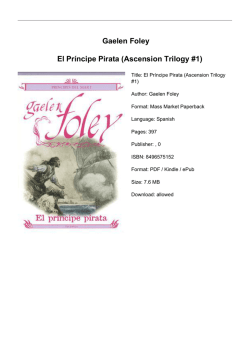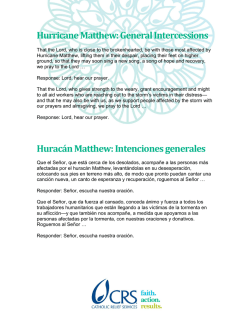# www.gwedynn.com/mates PROPORTION AND STATISTICS TEST

```PROPORTION AND STATISTICS TEST - 3º ESO
Exercise 1: (1.5 points) Determine if the following tables correspond to magnitudes directly or
inversely proportional, fill in the gaps and find the values of the constants of proportionality
2
6
9
5
12
0.5
1
3
12
15
10
6
15
12
18
20
3
0.75
Exercise 2: (1.25 points) The price of a house has increased by 12% this last year. If the price is
€90.000
a) What was the original price of the house?
b) I have to pay 21% of VAT and then pay 7.5% of interest to the bank. What's the final price of
the house?
Exercise 3: (1 point) Split €600 in an inversely proportional way to 2, 5 and 6.
Exercise 4: (1.25 points) Twenty workers need to work eight hours a day for nine days to finish
a job. How many hours a day would fifteen workers have to work to finish the same job in
twenty days?
Exercise 5: (1 point) Quiero estudiar la tasa de mortalidad (en porcentaje) entre los rebaños de
ovejas de la sierra. Para ello le he pedido a un pastor que haga un seguimiento de sus animales
durante un año y anote cuántos de ellos la han palmado. Digo, han fallecido. Sniff, sniff.
Clasifica la variable aleatoria e indica la población y la muestra. ¿Crees que es significativa?
¿Por qué?
Exercise 6: (2 points) Given the following table showing the values and frequencies of a certain
random variable
xi
0
4
7
10
20
fi
5
2
3
4
7
Work out:
a) The percentages
b) The range and the standard deviation
c) Do you think that I should repeat the study? Why?
Exercise 7: (2 points) Given the following table showing the values and frequencies of a certain
random variable
xi
[0,3]
(3,6]
(6,9]
(9,12]
(12,15]
fi
7
4
7
3
6
Work out:
a) Classify the variable
b) The measures of central tendency
c) Pearson's coefficient of variation
d) The frequency polygon
www.gwedynn.com/mates
```# Gaelen Foley El Príncipe Pirata (Ascension Trilogy #1)